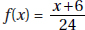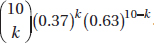# AP Statistics Multiple-Choice Practice Questions: Probability as Relative Frequency 4

### Test Information12 questions28 minutes

1. Suppose you are one of 7.5 million people who send in their name for a drawing with 1 top prize of \$1 million, 5 second-place prizes of \$10,000, and 20 third-place prizes of \$100. Is it worth the \$0.49 postage it cost you to send in your name?

2. You have a choice of three investments, the first of which gives you a 10% chance of making \$1 million, otherwise you lose \$25,000; the second of which gives you a 50% chance of making \$500,000, otherwise you lose \$345,000; and the third of which gives you an 80% chance of making \$50,000, otherwise you make only \$1,000. Assuming you will go bankrupt if you don't show a profit, which option should you choose for the best chance of avoiding bankruptcy?

3. Can the function, for x = 1, 2, and 3, be the probability distribution for some random variable?

4. Sixty-five percent of all divorce cases cite incompatibility as the underlying reason. If four couples file for a divorce, what is the probability that exactly two will state incompatibility as the reason?

5. Suppose we have a random variable X where for the values k = 0, ..., 10, the associated probabilities are. What is the mean of X?

6. A computer technician notes that 40% of computers fail because of the hard drive, 25% because of the monitor, 20% because of a disk drive, and 15% because of the microprocessor. If the problem is not in the monitor, what is the probability that it is in the hard drive?

7. Suppose you toss a fair coin ten times and it comes up heads every time. Which of the following is a true statement?

8. A city water supply system involves three pumps, the failure of any one of which crashes the system. The probabilities of failure for each pump in a given year are 0.025, 0.034, and 0.02, respectively. Assuming the pumps operate independently of each other, what is the probability that the system does crash during the year?

9. Which of the following are true statements?

I.The histogram of a binomial distribution with p = 0.5 is always symmetric no matter what n, the number of trials, is.

II.The histogram of a binomial distribution with p = 0.9 is skewed to the right.

III.The histogram of a binomial distribution with p = 0.9 appears almost symmetric if n is very large.

10. Suppose that 60% of students who take the AP Statistics exam score 4 or 5, 25% score 3, and the rest score 1 or 2. Suppose further that 95% of those scoring 4 or 5 receive college credit, 50% of those scoring 3 receive such credit, and 4% of those scoring 1 or 2 receive credit. If a student who is chosen at random from among those taking the exam receives college credit, what is the probability that she received a 3 on the exam?

11. Given the probabilities P(A) = 0.4 and P(AB) = 0.6, what is the probability P(B) if A and B are mutually exclusive? If A and B are independent?

12. There are 20 students in an AP Statistics class. Suppose all are unprepared and randomly guess on each of ten multiple-choice questions, each with a choice of five possible answers. Which of the following is one run of a simulation to estimate the probability at least one student guesses correctly on over half the questions?